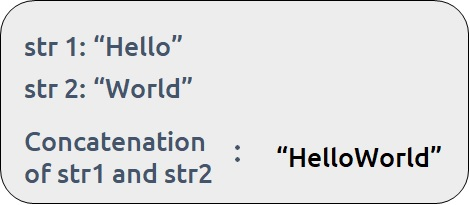# Concatenate one string to another

Problem Statement: Concatenate one string to another. Write a program to concatenate one string’s contents to another. Concatenation means to join two (or more) strings and form a combined string having the characters of both ( or all) strings in the same order as they were before in separate strings.

Examples:

``

Disclaimer: Don’t jump directly to the solution, try it out yourself first.

### Solution 1: Using the “+” operator

The “+” operator is designed in major programming languages ( including C++ and Java) such that when it is used with strings, it automatically concatenates them.

Code:

## C++ Code

``````#include <bits/stdc++.h>

using namespace std;

int main() {

string str1 = "Hello";
string str2= "World";

str1 = str1 + str2;

cout <<str1;

}
``````

Output:

HelloWorld

Time Complexity: O(1)

Space Complexity: O(1)

## Java Code

``````import java.io.*;
import java.util.*;

class takeuforward {

public static void main(String[] args)
{
String str1 = "Hello";
String str2 = "World";

str1 = str1+ str2;

System.out.print(str1);
}
}
``````

Output:

HelloWorld

Time Complexity: O(1)

Space Complexity: O(1)

Solution 2: Using library functions

Library functions in C++

The following inbuilt functions can be used to concatenate two strings in C++:

• strcat( )
• append()

# strcat()

The strcat()   function works on character arrays. It accepts a destination character array and a source character array and it concatenates them. It returns a character pointer to the concatenated character array pointed to the original destination array.

Note: The destination array should be large enough to include both the character arrays.

Syntax:

strcat ( char * destination, const char * source );

Code:

## C++ Code

``````#include <bits/stdc++.h>

using namespace std;

int main() {

char str1 ={'H','e','l','l','o'};
char str2= {'W','o','r','l','d'};

strcat(str1,str2);

cout<<str1;

}
``````

Output:

HelloWorld

Time Complexity: O(1)

Space Complexity: O(1)

# append()

The append() function is used to concatenate a string to another. This function works on string objects itself.

Syntax:

str1.append(str2);

Code:

## C++ Code

``````#include <bits/stdc++.h>

using namespace std;

int main() {

string str1 = "Hello";
string str2 = "World";

str1.append(str2);

cout<<str1;

}
``````

Output:

HelloWorld

Time Complexity: O(1)

Space Complexity: O(1)

Library function in Java

The following methods can be used to concatenate two strings in Java:

• concat()
• append() of StringBuilder class

### # concat()

The concat() method is used to concatenate two strings. It always returns a new string without modifying the existing string given as input.

Syntax:

str1.concat(str2);

## Java Code

``````import java.io.*;
import java.util.*;

class takeuforward {

public static void main(String[] args)
{
String str1 = "Hello";
String str2 = "World";

String str=str1.concat(str2);

System.out.print(str);
}
}
``````

Output:

HelloWorld

Time Complexity: O(1)

Space Complexity: O(1)

#append()

In concat() function, a new string is made first which is a time-costly operation. Therefore we can use the append() function of StringBuilder Class, which doesn’t make a new array(internal implementation of string) rather expands the already given array to save time.

Syntax:

stb1.append(stb2);

Code:

## Java Code

``````import java.io.*;
import java.util.*;

class takeuforward {

public static void main(String[] args)
{
String str1 = "Hello";
String str2 = "World";

StringBuilder stb1 = new StringBuilder(str1);

stb1.append(str2);

System.out.print(stb1);
}
}
``````

Output:

HelloWorld

Time Complexity: O(1)

Space Complexity: O(1)

### Solution 3: Using custom concat function

We can make our custom concatenation function which can be used to concatenate two strings. We will take a new string as a destination, to which the given two strings need to be concatenated.

Note: The destination array size needs to be big enough to store both strings. We will be using a character array representation of strings. In C++, every character array ends with a null character(‘\0’) so we need to add it explicitly after concatenation.

Approach:

We will iterate through the first string and copy the values to our destination string at each index. Next, we iterate the second string and continue to copy its value to our destination string. At the start of the second loop, we will use the index value for the destination string whatever was set after the first loop. This way concatenation happens by using that same index.

Code:

## C++ Code

``````#include <bits/stdc++.h>

using namespace std;

int main() {

char dest;

char str1= {'H','e','l','l','o'};
char str2= {'W','o','r','l','d'};

int i=0,j=0;

while(str1[i]!='\0'){
dest[i]=str1[i];
i=i+1;
}

while(str2[j]!='\0'){
dest[i]= str2[j];
i=i+1;
j=j+1;
}

dest[i]='\0';

cout<<dest;

}
``````

Output:

HelloWorld

Time Complexity: O(n+m), where n is the length of the first string and m is the length of the second string.

Space Complexity: O(n+m), where n is the length of the first string and m is the length of the second string.

## Java Code

``````import java.io.*;
import java.util.*;

class takeuforward {

public static void main(String[] args)
{
char[] str1 = {'H', 'e', 'l', 'l', 'o'};
char[] str2 = {'W', 'o', 'r', 'l', 'd'};

int n = str1.length;
int m = str2.length;

char[] dest = new char[n1+n2];

for(int i=0;i<n;i++){
dest[i] = str1[i];
}

for(int j=0; j<m; j++){
dest[j+n1]= str2[j];
}

System.out.print(new String(dest));
}
}
``````

Output:

HelloWorld

Time Complexity: O(n+m), where n is the length of the first string and m is the length of the second string.

Space Complexity: O(n+m), where n is the length of the first string and m is the length of the second string.

Special thanks to Anshuman Sharma for contributing to this article on takeUforward. If you also wish to share your knowledge with the takeUforward fam, please check out this article